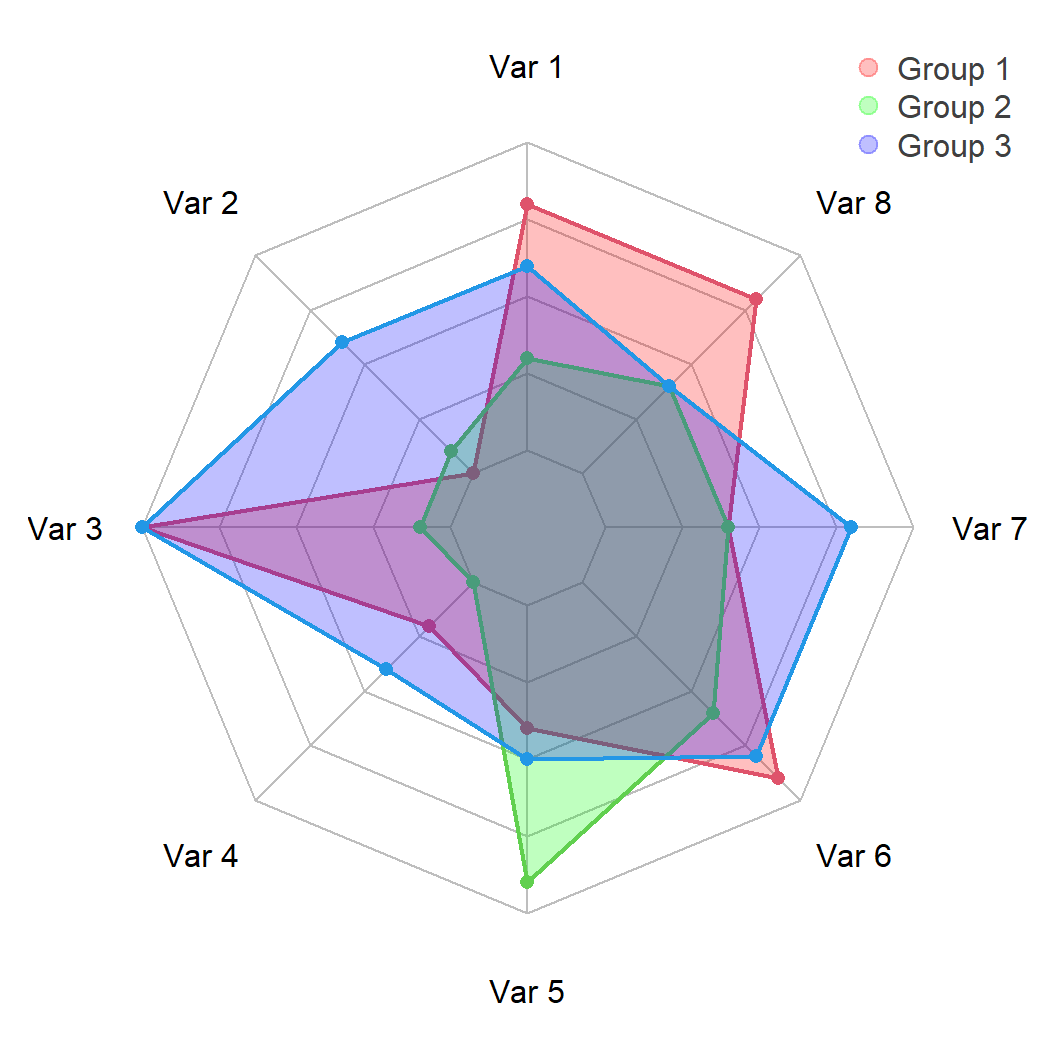# Radar chart (spider plot) in R with fmsb

Minato Nakazawa

## Sample data sets

The `radarchart` function from `fmsb` package needs a data frame where the first row represents the maximum values of the data (10) and the second row the minimum values (0). Otherwise, you will need to set `maxmin = FALSE`. Note that the number of columns must be greater than two.

Each column is a variable and the additional rows represents groups or individuals. In `df` we have only one group and 8 different variables.

Data set with one group

``````set.seed(1)
df <- data.frame(rbind(rep(10, 8), rep(0, 8),
matrix(sample(0:10, 8),
nrow = 1)))
colnames(df) <- paste("Var", 1:8)``````

If you want to add more groups or individuals append more rows to the data frame. In `df2` we have 8 variables of tree different groups.

Data set with several groups

``````set.seed(1)
df2 <- data.frame(rbind(rep(10, 8), rep(0, 8),
matrix(sample(0:10, 24,
replace = TRUE),
nrow = 3)))
colnames(df2) <- paste("Var", 1:8)``````

The default radar chart can be created passing the data frame to the `radarchart` function of the package.

``````# install.packages("fmsb")
library(fmsb)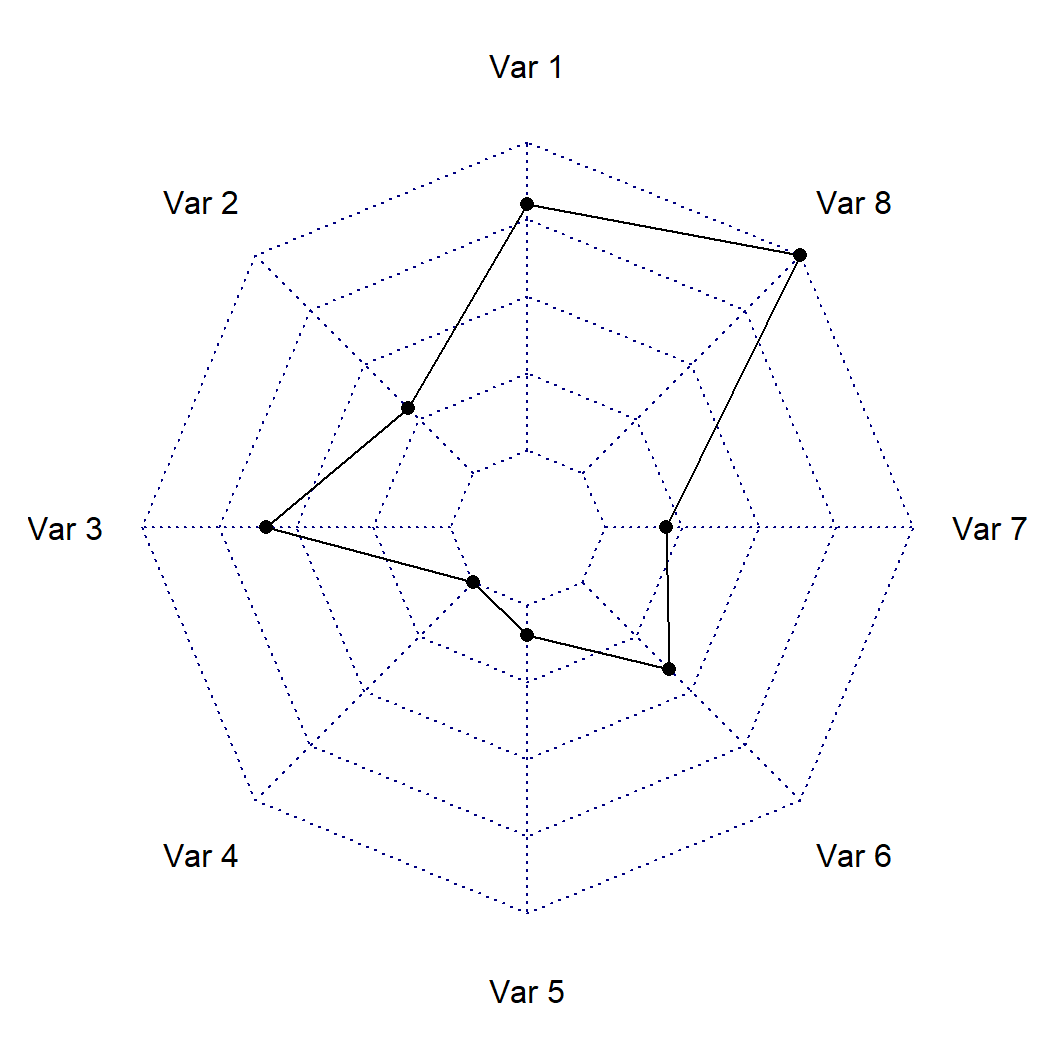If you don’t like the default styling of the plot you can customize it. The `cglty`, `cglcol` and `cglwd` arguments can be used to change the line type, color and line width of the grid lines and `pcol`, `plwd` and `plty` to change the color, line width and linetype of the line representing the values of the variables.

``````# install.packages("fmsb")
library(fmsb)

cglty = 1,       # Grid line type
cglcol = "gray", # Grid line color
cglwd = 1,       # Line width of the grid
pcol = 4,        # Color of the line
plwd = 2,        # Width of the line
plty = 1)        # Line type of the line``````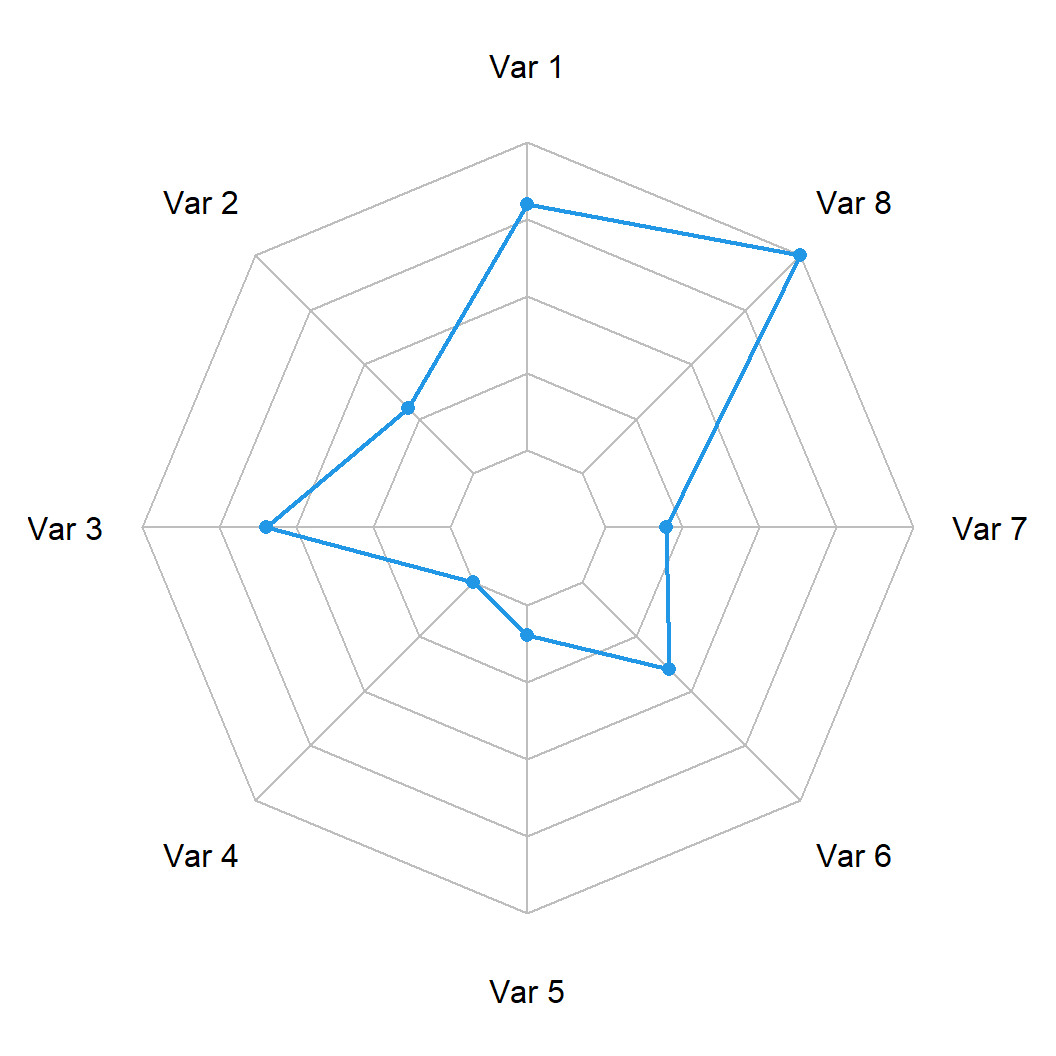## Fill the area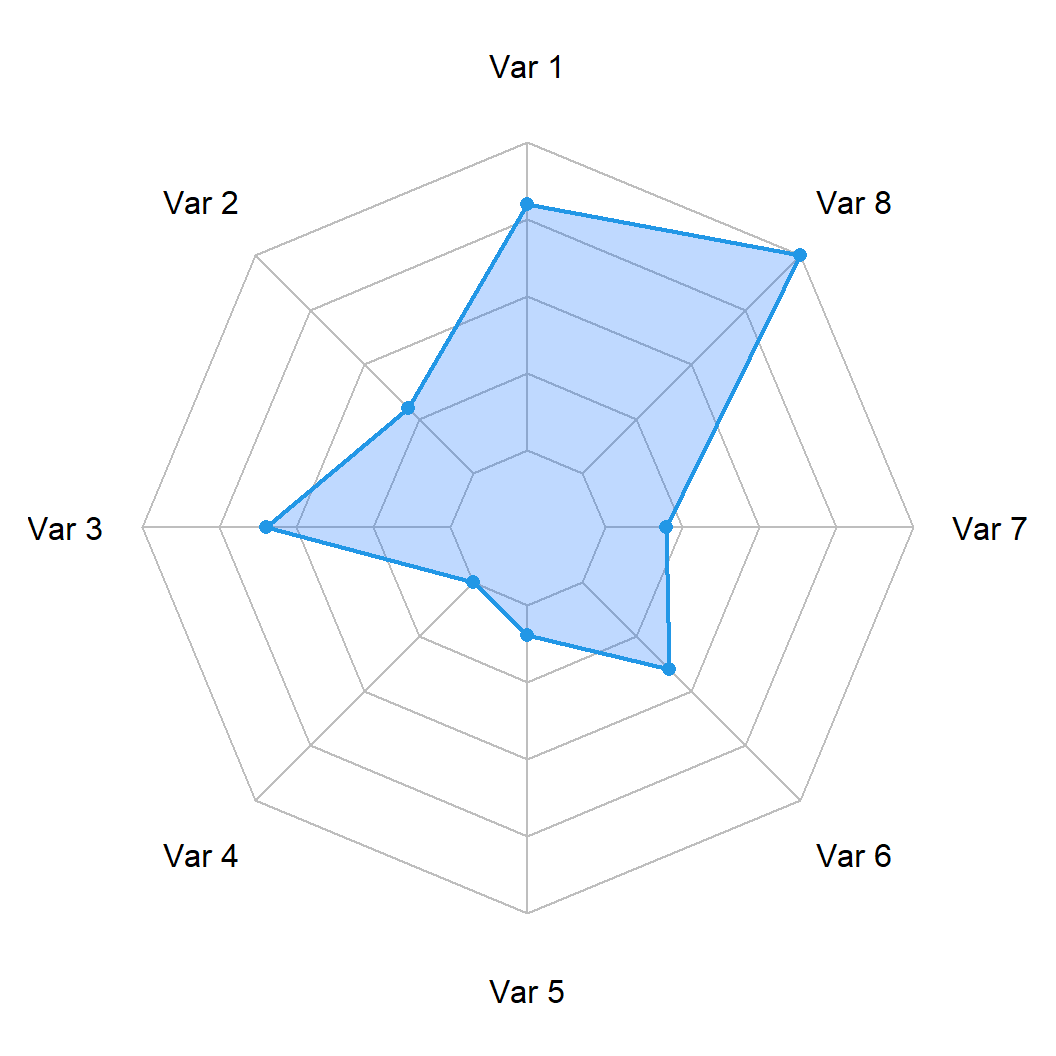You can also fill the area of the polygon created making use of the `pfcol` argument. It is recommended to use a color with transparency for a better result.

``````# install.packages("fmsb")
library(fmsb)

cglty = 1, cglcol = "gray",
pcol = 4, plwd = 2,
pfcol = rgb(0, 0.4, 1, 0.25))``````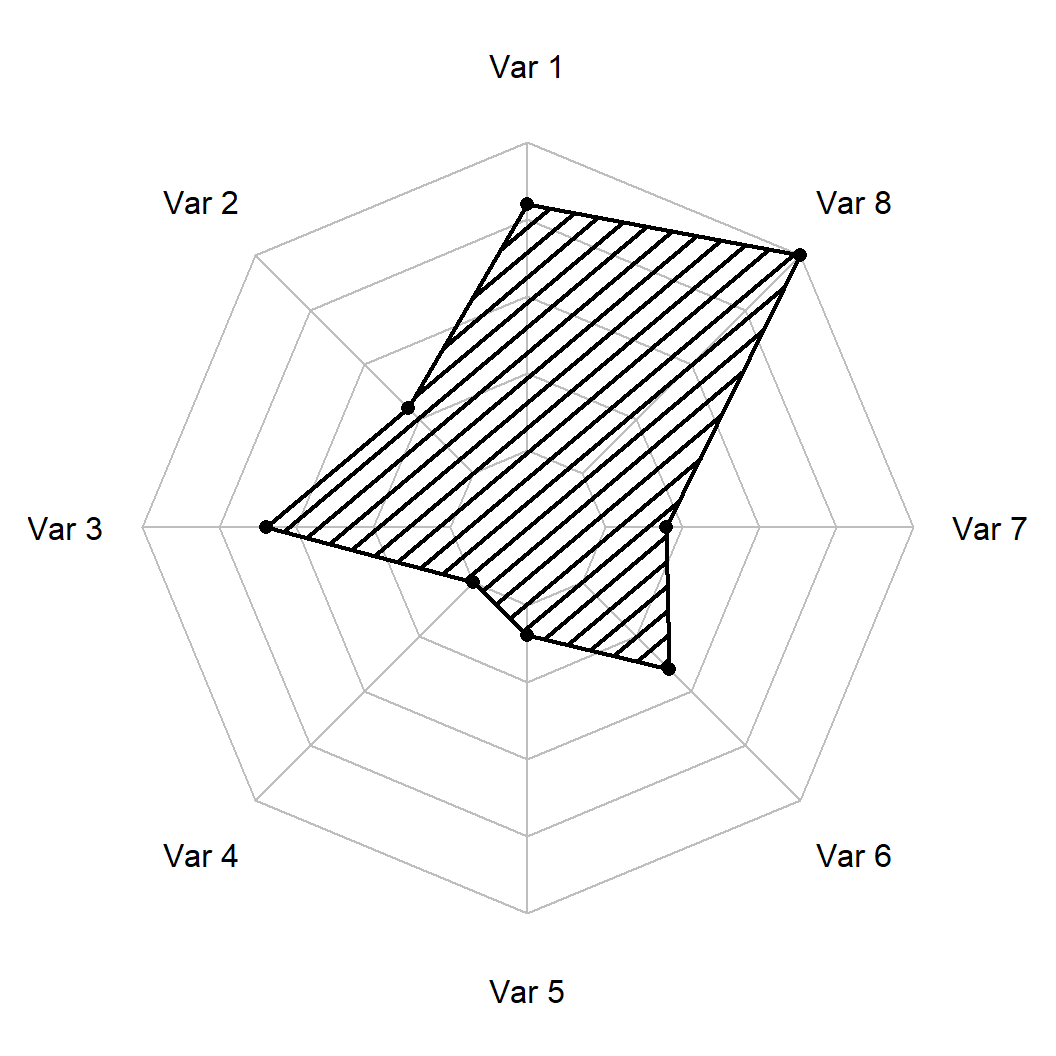Alternatively, you can add shading lines to the polygon with `pdensity` and change their angle with `pangle` argument.

``````# install.packages("fmsb")
library(fmsb)

cglty = 1, cglcol = "gray",
pcol = 1, plwd = 2,
pdensity = 10,
pangle = 40) ``````

## Radar chart of several groups

The process for creating a radar chart for several groups or individuals is the same as in the previous section, you just need to pass the data frame with several variables we created on the first section.

``````# install.packages("fmsb")
library(fmsb)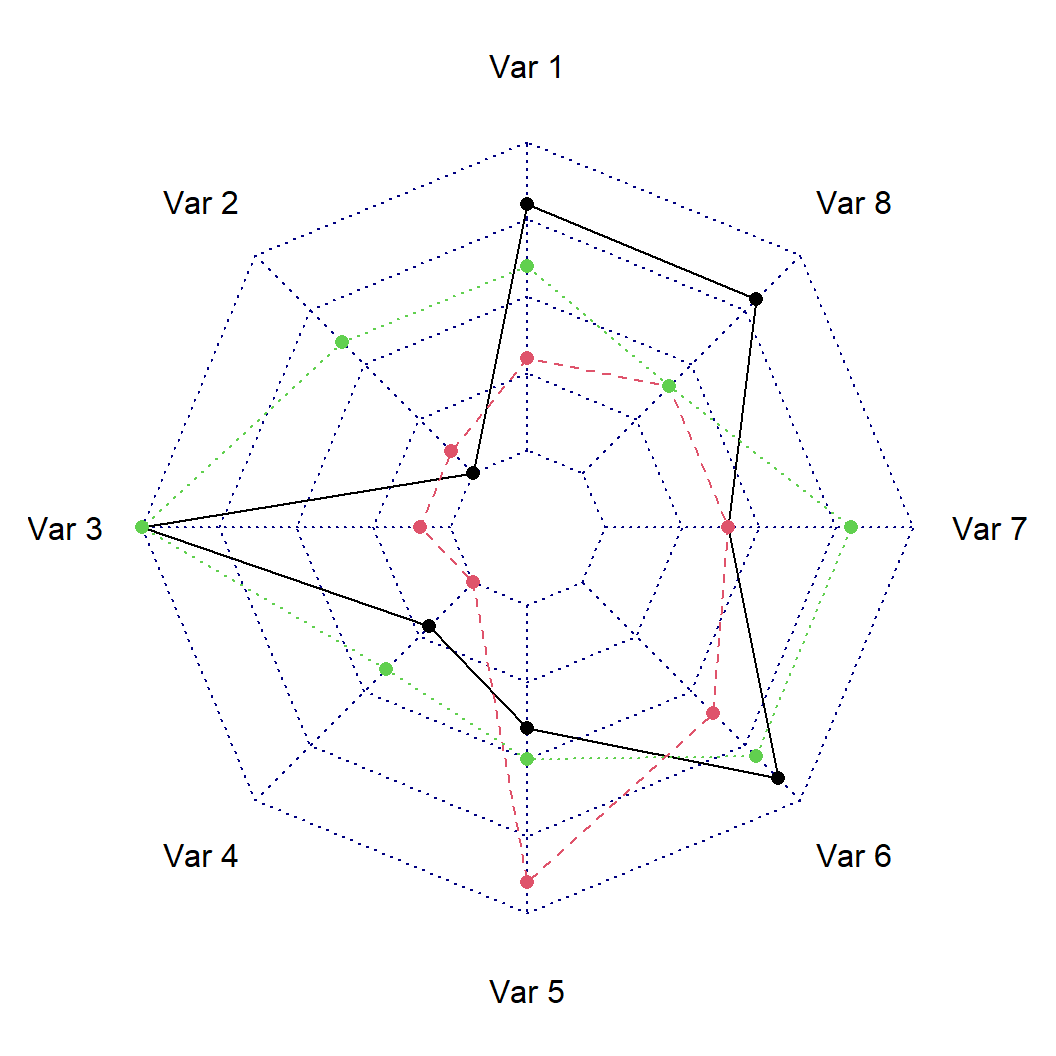Lines customization

In this scenario you can customize the colors, line styles and line widths for each group.

``````# install.packages("fmsb")
library(fmsb)

cglty = 1,       # Grid line type
cglcol = "gray", # Grid line color
pcol = 2:4,      # Color for each line
plwd = 2,        # Width for each line
plty = 1)        # Line type for each line ``````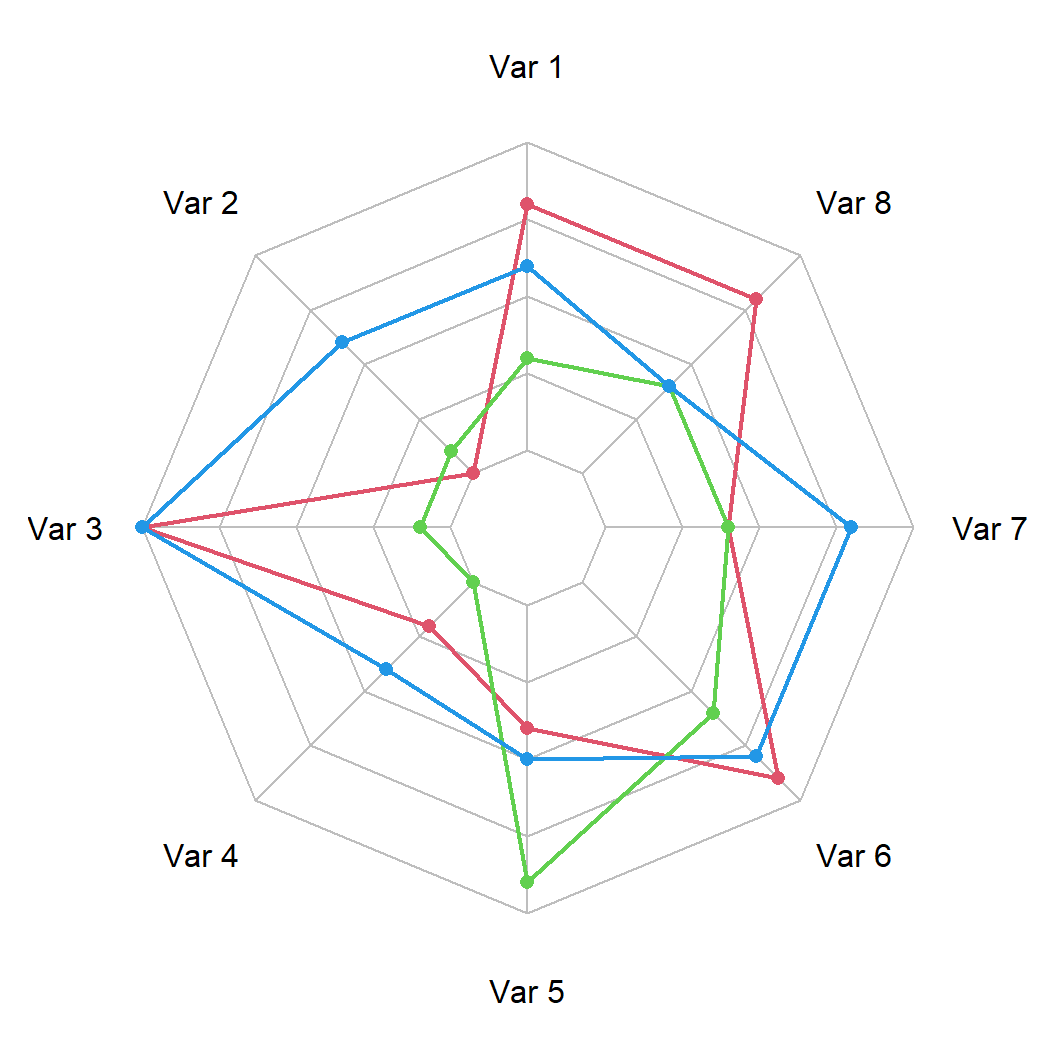Fill areas

You can also set a fill color for each group.

``````# install.packages("fmsb")
library(fmsb)

# Fill colors
areas <- c(rgb(1, 0, 0, 0.25),
rgb(0, 1, 0, 0.25),
rgb(0, 0, 1, 0.25))

cglty = 1,       # Grid line type
cglcol = "gray", # Grid line color
pcol = 2:4,      # Color for each line
plwd = 2,        # Width for each line
plty = 1,        # Line type for each line
pfcol = areas)   # Color of the areas  ``````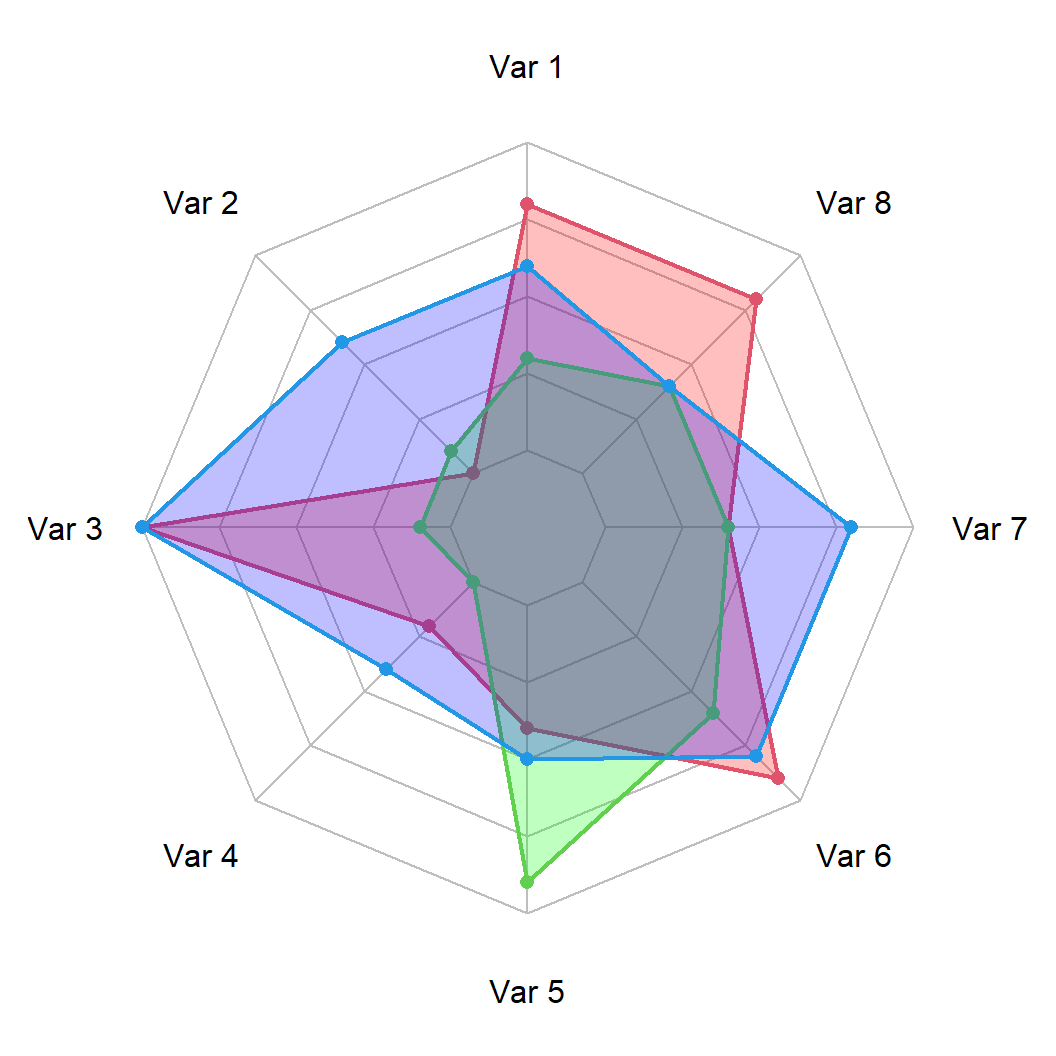Finally, it is recommended to add a legend when displaying several groups making use of the `legend` function, as shown in the following example.

``````# install.packages("fmsb")
library(fmsb)

areas <- c(rgb(1, 0, 0, 0.25),
rgb(0, 1, 0, 0.25),
rgb(0, 0, 1, 0.25))

cglty = 1,       # Grid line type
cglcol = "gray", # Grid line color
pcol = 2:4,      # Color for each line
plwd = 2,        # Width for each line
plty = 1,        # Line type for each line
pfcol = areas)   # Color of the areas

legend("topright",
legend = paste("Group", 1:3),
bty = "n", pch = 20, col = areas,
text.col = "grey25", pt.cex = 2)``````